## conference papersJOURNAL OFAPPLIEDCRYSTALLOGRAPHY
ISSN: 1600-5767
Volume 40| Part s1| April 2007| Pages s200-s204

## Shape of α-crystallin analyzed by small-angle neutron scattering

aResearch Reactor Institute, Kyoto University, Osaka 590-0494, Japan, bHigh Energy Accelerator Research Organization, Ibaraki 305-0801, Japan, cInstitute of Natural Science and Technology, Niigata University, Niigata 950-2181, Japan, and dDepartment of Chemistry, Kyushu University, Fukuoka 812-8581, Japan
*Correspondence e-mail: sugiyama@rri.kyoto-u.ac.jp

(Received 16 August 2006; accepted 8 February 2007; online 6 April 2007)

The size and shape of aggregates of human recombinant αA-crystallin and αB-crystallin are investigated with small-angle neutron scattering and dynamic light scattering. At a bioactive temperature (310 K), both polypeptides form aggregates with almost the same size and shape. The αB-crystallin maintains an almost identical size and shape at 310 and 288 K, whereas the aggregate of αA-crystallin shows deformation at 288 K. This result suggests that at the lower temperature there is a difference in structural stability between the two aggregates of the polypeptides.

### 1. Introduction

It is well known that the function of a protein is deeply related to its three-dimensional structure: a regular structure generates a regular function. In other words, when a protein loses its regular structure by misfolding and so on, it does not work regularly. The irregular protein is acknowledged and then repaired or broken down into its amino acids. However, certain irregular proteins aggregate and then accumulate in an organism. In the long term, the accumulation of these abnormal aggregates can cause serious disease, such as variant Creutzfeldt–Jakob disease (by abnormal prion), Alzheimer's disease (by β-amyloid proteins), and so on.

We have two questions about the aggregation of these abnormal proteins: what is the trigger of an abnormal aggregation and how does the aggregation proceed? In order to answer these questions, we have looked at a human eye lens protein, crystallin: under environmental stresses, such as UV, X-ray, γ-ray irradiation and low temperature, crystallin makes huge aggregates. When the abnormal aggregates accumulate in a human eye lens, a cataract finally develops.

There are three types of crystallins in the human eye lens, α, β and γ-crystallins. Among them, α-crystallin plays an important role in preventing the crystallins from making the abnormal aggregation with its chaperone activity. In fact, as the aggregation of α-crystallin progresses abnormally, insoluble aggregates with high molecular weight (HMW; more than 106 Da) appear. In an aged and cataractous lens, a large quantity of HMW aggregates has been observed. Therefore, it is considered that α-crystallin is a key protein to solve the questions above.

The trigger of abnormal aggregation of α-crystallin is not completely known, but it may be related to post-translational modifications such as deamidation (Voorter et al., 1988), racemization and isomerization (Fujii, Satoh et al., 1994; Fujii, Ishibashi et al., 1994), truncation (Miesbauer et al., 1994; Emmons & Takemoto, 1992; Takemoto, 1995), phosphorylation (Miesbauer et al., 1994), oxidation (Takemoto, 1996a), an increase in intramolecular disulfide bonding (Takemoto, 1996b; Cherian-Shaw et al., 1999) and glycation (Swamy et al., 1993). Racemization and isomerization of amino acids in protein can cause major changes in structure, since different side-chain orientations can induce an abnormal peptide backbone. Truncated subunits, the increase of intramolecular disulfide bonding and advanced glycated end-products may also perturb the normal closed-packing structure for the crystallins. Therefore, these post-translational modifications can induce the partial unfolding, resulting in a reduction of chaperone-like activity, followed by the eventual formation of cataracts.

The structural information of native and abnormal aggregates is important to reveal the abnormal aggregation process of human α-crystallin after undergoing the post-translational modifications. However, the structural characterization of those aggregates such as size and shape, has not yet been sufficient. For the structural investigation of α-crystallin, the first problem is obtaining enough of the human α-crystallin and the second is that the single-crystal X-ray structure analysis method, which is a most powerful tool, cannot be utilized since the crystallization of α-crystallin has not been achieved so far. In our work, human recombinant αA- and αB-crystallins, which are subunits consisting of α-crystallin, were expressed in BL21(DE3)pLysS Escherichia coli cells. In addition, small-angle neutron scattering (SANS) and dynamic light scattering (DLS) methods are adopted to observe the size and shape of the aggregates of these subunits since both methods can reveal structural information about the sample in a solution.

In this paper, we firstly report the shape and size of the aggregates consisting of human recombinant αA- and αB-crystallins and their structural stability depending upon the temperature.

### 2. Experimental

#### 2.1. Sample preparation

In its native state, α-crystallin is a water-soluble aggregate with a molecular weight of approximately 800 kDa. The aggregate is comprised of two kinds of subunits, αA- and αB-crystallins with 173 and 175 amino-acid residues, respectively. Since the molecular weight of both subunits is approximately 20 kDa, the native aggregate of α-crystallin contains approximately 40 subunits. We prepared human αA- and αB-crystallins for expression by E. coli as described in the next section. After purification and condensation, light water solutions of αA-crystallin and αB-crystallin were used for DLS measurements. In addition, after exchanging the solvent (light water) with heavy water, SANS experiments were conducted with those D2O solution samples.

##### 2.1.1. Overexpression of human recombinant αA- and αB-crystallins

A DNA fragment containing human αA-crystallin was obtained by polymerase chain reaction (PCR) amplification from first-stranded cDNAs of human fetal brain (Clontech, CA) with a sense primer of 5′-CCATGGACGTGACCATCCAG-3′ and an antisense primer of 5′-GGCTGCTATCTAAAGGAGT-3′. The sense primer was designed to include the NcoI site and the italics in the nucleotide sequence shows the restriction site. The PCR reaction was carried out for 40 cycles of denaturation (367 K, 30 s), annealing (333 K, 30 s) and extension (345 K, 60 s). The PCR product was gel-purified and subcloned using a pBluescript TA vector (Strategene, CA). The NcoI–BamHI digestion product of the DNA fragment of αA-crystallin was ligated into the NcoI–BamHI site of the T7 expression vector pET-3d (Novagen, WI).

Recombinant human αB-crystallin cDNA, a gift from J. Mark Petrash (Washington University), was subcloned into the NcoI/HindIII sites of the vector pET-23d(+) (Novagen).

αA- and αB-crystallin plasmids were inserted into the E. coli strain BL21(DE3)pLysS, and expressed as recombinant αA- and αB-crystallins. E. coli transformants were cultured for 12 h in 10 ml LB culture solution containing 50 µg ml−1 ampicillin and 30 µg ml−1 chloramphenicol. After 12 h, 10 ml culture solution was added to 1 l LB, and cultured for approximately 2 h (OD600 = 0.6), after which the expression of αA- and αB-crystallins was induced by addition of isopropyl-1-thio-β-D-galactopyranoside (IPTG) to a final concentration of 0.3 mM and growth for an additional 5 h at 298 K. After IPTG induction, E. coli cells were collected by centrifugation and sonicated in 50 mM Tris–HCl buffer pH 7.8, 100 mM NaCl, 1.0 mM EDTA, 1.0 mM phenylmethanesulfonyl fluoride. Sonicated preparations were separated into the soluble fraction and pellets by centrifugation. The soluble fraction, including the proteins, was placed in an ion-exchange column (Q Sepharose Fast Flow, Amersham Biosciences) and eluted using a gradient of 0–1 M NaCl at 3.0 ml min−1. αA- and αB-crystallins were recovered in 200–300 mM and 100–200 mM NaCl fractions, respectively. Subsequently, the samples were applied to a gel filtration HPLC column (TSK gel-G3000SW, Tohso, Japan). The void volume fraction was collected and concentrated using an Amicon-Ultra 4 column.

##### 2.1.2. SDS–PAGE and Western blot analysis of recombinant human αA- and αB-crystallins

Recombinant human αA- and αB- crystallins were expressed in E. coli. Purified crystallins were dissolved in sample buffer (2% SDS, 6% 2-mercaptoethanol, 10% glycerol, 0.05 M Tris–HCl pH 6.8) and analyzed by SDS–polyacrylamide gel electrophoresis (SDS–PAGE). Separated proteins were detected by Coomassie brilliant blue stain. For Western blot analysis, the proteins were loaded and run on a 15% SDS–polyacrylamide gel, transferred onto a polyvinylidene difluoride membrane, blocked with 5% dried milk in PBS buffer, and immuno­blotted with anti αA- or αB-crystallin antibodies followed by goat anti-rabbit horseradish peroxidase-conjugated secondary antibody. Immunoreactive bands were visualized by ECL chemiluminescent detection (Amersham Biosciences).

#### 2.2. Dynamic light scattering

ALV DLS/SLS-5000 light scattering system equipped with an ALV-5000 multiple digital correlation with light source from a Uniphase 22 mW He–Ne laser (λ = 632.8 nm) was used for the DLS experiments. The experiments were performed at seven different scattering angles (30, 45, 60, 90, 120, 135 and 150°) at 310 K. The sample concentrations of αA- and αB-crystallin were tuned to be 1.0 mg ml−1.

#### 2.3. Small-angle neutron scattering

SANS experiments were performed with D2O solutions of αA- and αB-crystallin at 310 K and 288 K using the small/wide-angle neutron diffractometer (SWAN) installed at the spallation neutron source of Neutron Science Laboratory (KENS), Institute of Material Structure Science, High Energy Accelerator Research Organization KEK, Japan. The SANS intensity of the sample solution was corrected following the standard correction for transmission, background scattering, cell scattering and buffer solution scattering, and then the scattering from the solute (protein) could be obtained. The sample concentration was 3.0 mg ml−1 and the measurement time was 6 h for both samples.

### 3. Analysis

#### 3.1. Dynamic light scattering

A intensity–intensity time correlation function g(2)(Γ) is measured by a DLS spectrometer: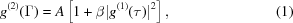where A, β and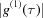are the background, a coherence factor and normalized electric field correlation function, respectively (Brown, 1996). The electric field correlation function was analyzed by the constrained regularized CONTIN method (Provencher et al., 1978), to yield information on the distribution of the characteristic line width G(Γ) from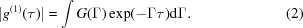The normalized distribution function of the characteristic line width G(Γ) thus obtained can be used to determine an average apparent diffusion coefficient Dav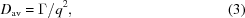where q = (4πn/λ)sin(θ/2) is magnitude of the scattering vector, n is the refractive index of the solvent, λ is the wavelength of the incident light, θ is the scattering angle. Within the dilute regime, Dav varies linearly with the concentration according to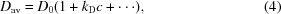where D0 is diffusion coefficient at infinite dilution and kD is hydrodynamic virial coefficient. The Stokes–Einstein equation relates D0 to the apparent hydrodynamic radius Rh: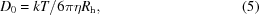where k is Boltzmann's constant and η is viscosity of water at temperature T.

#### 3.2. Small-angle neutron scattering

In order to find a radius of gyration Rg, we conducted a Guinier approximation in the low-q region: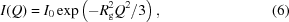where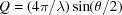is the magnitude of scattering vector, λ and θ are the incident neutron wavelength and the scattering angle, respectively. The scattering intensity is shown as a straight line and the slope of line indicates Rg2/3 on Guinier plot ln[I(Q)] versus Q2.

It is worthwhile looking at the distance distribution function P(R) to find the best comparable shape. The distance distribution function P(R) is given by the Fourier transformation of scattering intensity I(Q) as follows,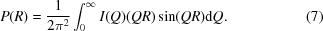To reduce the termination error on the Fourier transformation, SWAN is a very suitable spectrometer since it can observe a scattering intensity in a wide Q-range (10−3 to 100 Å−1) utilizing with features of a time-of-flight spectrometer and a pulsed neutron source at the same time.

### 4. Results and discussion

#### 4.1. Size and shape at a bioactive temperature (310 K)

To start with we will examine the result of DLS measurements. Fig. 1shows the observed intensity–intensity time correlation and the distribution of characteristic line width of αA- and αB-crystallin solutions at θ = 60° (q = 1.87 × 107 m−1). Only a sharp single peak is observed in each of G(Γ) plots. Therefore, in each sample, an aggregate is almost monodispersing and there is no aggregate with the higher molecular weight.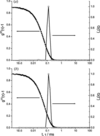Figure 1 Typical results of DLS measurements of (a) αA-crystallin solution and (b) αB-crystallin solution. Filled and open circles denote the intensity–intensity time correlation g(2)(Γ) and the distribution of characteristic line width G(Γ) at θ = 60° (q = 1.87 × 107 m−1), respectively. Both panels indicate that the particles are monodispersed and there is no huge aggregate in the solution.

Fig. 2shows the angular dependence of the relaxation rates (Γ versus q2) of αA- and αB-crystallin aggregates. The relaxation rate Γ is proportion to q2, as expected for diffusive motion of a monodisperse particle [equation (3)]. Average diffusion constants for αA- and αB-crystallin aggregates are found to be 4.74 ± 0.17 × 10−11 and 4.42 ± 0.17 × 10−11 m2 s−1, respectively. Assuming that the concentration is low, the hydrodynamic radii Rh of αA- and αB-crystallin aggregates are 69.6 ± 2.5 and 74.7 ± 2.9 Å from equations (4)and (5), respectively; the results suggest that the size of the aggregate of αA-crystallin could be slightly smaller than that of αB-crystallin.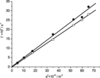Figure 2 Angular dependence of the relaxation rates of two aggregates at 310 K. Filled and open circles indicate those of αA- and αB-crystallin, respectively. The straight lines show the results of the least-squares fitting with equation (3).

SANS gives us more direct information of particle size in solution. Fig. 3shows scattering intensities (inset figures) and their Guinier plots of αA- and αB-crystallins at 310 K. As shown in the figure, the Guinier approximation is observed well in this Q-range. From equation (6), the Rg values of αA- and αB-crystallins are found to be 51.6 ± 0.2 and 52.4 ± 0.1 Å, respectively. The size relation between two aggregates is consistent with that resulting in the DLS experiments: the aggregate of αA-crystallin is slightly smaller than that of αB-crystallin. The ratios of two characteristic radii ρ (= Rg/Rh) are 0.74 ± 0.03 and 0.70 ± 0.03 for the αA- and αB-crystallin aggregates, respectively. Since ρ is 0.78 for a sphere, it could be said that the aggregates of both crystallins have a shape similar to a sphere.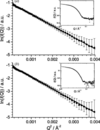Figure 3 Guinier plots and SANS profiles (inset figures) of the (a) αA-crystallin and (b) αB-crystallin aggregates at 310 K. The straight lines show the results of the least-squares fitting with equation (6).

To analyze the more detailed structure, we calculated a distance distribution function P(R) with equation (7). The scattering intensity at less than 0.01 Å−1 was extrapolated with the Guinier formula [equation (6)]. Moreover, the observed scattering intensity was used until the high Q-range (to 1.0 Å−1) in order to reduce the termination error on Fourier transformation. Accordingly, the resolution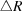on P(R) is theoretically given by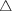R = 2π/Qmax ≃ 6 Å.

Fig. 4shows P(R) for the αA- and αB-crystallin aggregates at 310 K. Both sets of P(R) are good agreement with each other indicating that the shapes of the αA- and αB-crystallin aggregates are almost same. However, an R-section on P(R) of the αB-crystallin aggregate (∼160 Å) is slightly longer than that of αA-crystallin aggregate (∼145 Å). This is also consistent with the difference of Rg between two aggregates as described before.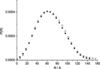Figure 4 Distance distribution functions P(R) calculated with equation (7) at 310 K. Filled and open circles denote P(R) of the αA- and αB-crystallin aggregates, respectively.

Considering that ρ ratios of both aggregates are close to those of a rigid sphere, we assumed that the shapes of the αA- and αB-crystallin aggregates could be a slightly elongated sphere. As the first approximation, we supposed that the shape could be a rotational body of the following equation,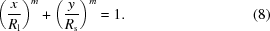Here, Rl and Rs are half distances of the longest and the shortest axes, respectively, m is a control parameter and the rotational axis is the x axis. For example, shapes are spheroid and cylinder at m = 2 and, respectively. We found the best compromised R and m by comparing P(R) following equation (8)with an observed P(R): Rl is obtained from the R-section (see Fig. 4). Under the rotational body assumption, the best compromised ellipticity r (= Rs/Rl) and m are 0.70 and 3.0 for the αA-crystallin aggregate, and 0.65 and 2.8 for the αB-crystallin aggregate. As shown in Fig. 5, P(R) calculated with the rotational body model reproduces well the observed ones.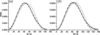Figure 5 Calculated and observed distance distribution functions P(R) of (a) the αA-crystallin and (b) the αB-crystallin aggregates. Filled circles show the observed P(R). The solid curves indicate P(R) calculated from the best compromised shapes based on the rotational body assumption (see text). The dash curves represent P(R) of a rigid sphere with a radius R corresponding to an observed Rg: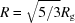.

#### 4.2. Structural deformation in low temperature (288 K)

Fig. 6shows scattering intensities (inset figures) and the Guinier plots of the αA- and αB-crystallin aggregates at 288 K. From equation (6), Rg of the αA- and αB-crystallin aggregates at 288 K are found to be 48.0 ± 0.2 and 51.6 ± 0.2 Å, respectively. This result indicates that the αA-crystallin aggregate changes its size whereas the αB-crystallin aggregate maintains it in cooling from 310 to 288 K.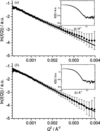Figure 6 Guinier plots and SANS profiles (inset figures) of the (a) αA-crystallin and (b) αB-crystallin aggregates at 288 K. The straight lines show the results of the least-squares fitting with equation (6).

The structural change of the aggregate of αA-crystallin in cooling could be more clearly exhibited in the distance distribution function P(R). Fig. 7shows P(R) of the αA- and αB-crystallin aggregates at 288 and 310 K. P(R) of the αA-crystallin aggregate at 288 K shows a remarkably different profile from the others: the peak position is shifted from 60 to 54 Å. From the decrease of Rg and the change of P(R) profile, it could be said that the aggregate of αA-crystallin is more deformed than that of αB-crystalline by cooling (310 K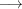288 K).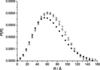Figure 7 Distance distribution functions P(R) calculated with equation (7). Filled circles and open circles denote data for αA- and αB-crystallin at 288 K and + and × denote data for αA- and αB-crystallin at 310 K, respectively.

The identity of amino acid sequence is more than 60% between αA- and αB-crystallins. Therefore, it is reasonable that under a bioactive condition (310 K) the sizes and shapes of the αA- and αB-crystallin aggregates are almost identical as shown in Figs. 3and 4. The question naturally follows: why does α-crystallin need two types of the comparable subunits to make the bioactive aggregate? One possible answer is that α-crystallin uses two polypeptides with different susceptibility against externally induced damages, such as UV radiation and low temperature, to have the resistance against those external stresses. In fact, Liao et al. (2002) showed that there was a different susceptibility between αA- and αB-crystallin against UV and thermally-induced damage by measuring their chaperone activities: they pointed out that αA-crystallin is a better chaperon against UV irradiation than αB-crystallin and that αB-crystallin is generally a better chaperoning protein than αA-crystallin at physiological temperatures. Recent ultracentrifugation experiments reported that αA-crystallin shows larger mass variation than αB-crystallin at low temperature (Fujii, 2006, private communication). Concerning the structural deformation of the αA-crystallin aggregate at a low temperature as shown in Fig. 7, this study also supports the result of ultracentrifugal analysis. Here it is very important to reveal the difference in susceptibility between αA- and αB-crystallins against UV irradiation from the structural points of view. Therefore, the SANS investigation for the UV-irradiated aggregates of two polypeptides is now in progress.

### Acknowledgements

This work was partly supported by Grant-in-Aid for Creative Scientific Research (No. 16GS0417 to MS). The DLS experiments were also supported by a Grant-in-Aid (No. 17350060) from the Ministry of Education, Culture, Sports, Science, and Technology (for MA).

### ReferencesJOURNAL OFAPPLIEDCRYSTALLOGRAPHY